Gujarati Lesson 2

Lesson 2

Lesson 3 ..Home
This is the way that the vowels are written on their own .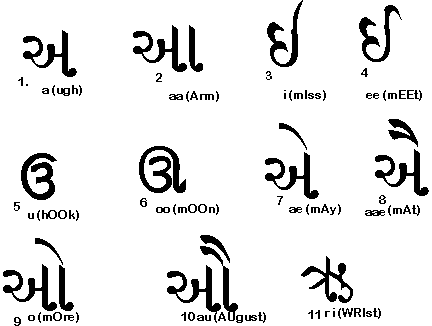Notice that each has a number . See if you can match each detached vowel with its corresponding vowel mark in the figure below.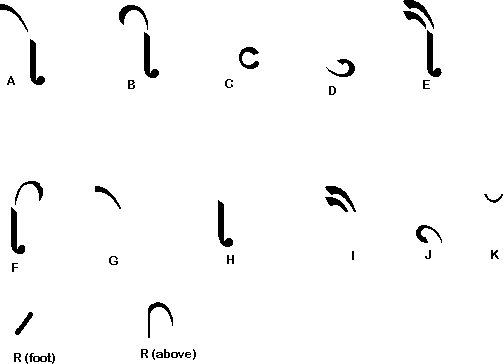The answers are 1K , 2H, 3F, 4B, 5D , 6J, 7G, 8I , 9A, 10 E
Note the accent mark over a letter as in K in the above figure . Note also the short diagonal R put in the foot of a letter . It converts the letter F in Fan for example to Fr in France as we shall see later. Rarely used is another R mark above a letter as the last item in the figure above. It is useful to know this, as some classical Gujarati is written in the Devanagri script and this kind of R mark above a letter is sounded before the letter - for example in the word Mark it would be put above K . Can you identify the following mixture of gujarati and English words ?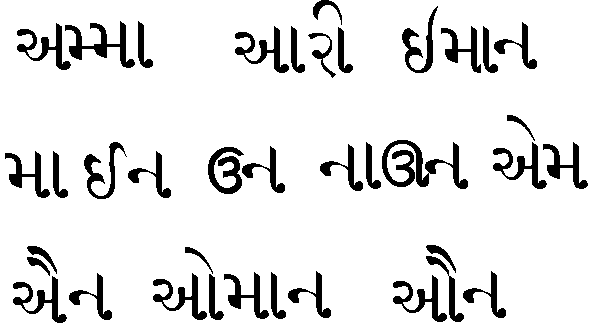The answers are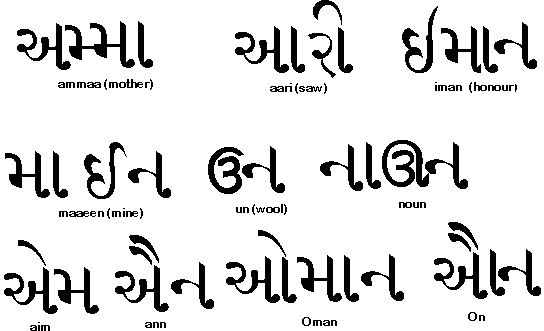. Note as in Hindi one has half letters , in this case M in Amma.

Now we look at the guttrals- so called because they are sounded from the back of the thorat -the first line in the Gujarati alphabet.Once gain below them in the next figure are the previously learned letters M , N and R and CHH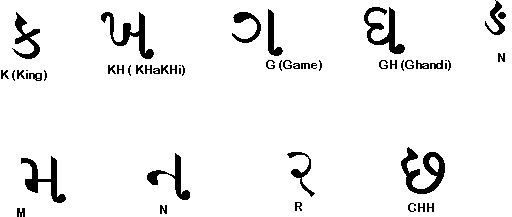In the next lesson we shall try to form sentences with all the letters learned so far. Lesson 3 ..Home